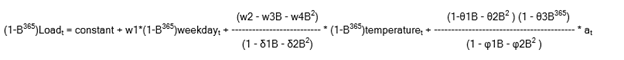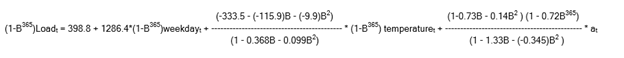## Issues Constructing ARIMA Model from Output file

Hey, all. New to SAS and having some issues trying to reconstruct an ARIMA equation, as I am having difficulty in deciphering the meaning behind some of these values and abbreviations. I have attached the outest file from Forecast Studio here, as well as a snippet. I am not well versed in the actual code behind SAS and it's GUIs. Any information regarding how best to read and/or construct the equation (if possible) is greatly appreciated.3 REPLIES 3

## Re: Issues Constructing ARIMA Model from Output file

ARIMA and ARIMAX models are often expressed using backshift notation.  The structure of the OUTEST data set generated by Forecast Studio makes it convenient to reconstruct the model using backshift notation.

A description of the variables in the OUTEST data set created by Forecast Studio can be found at the following documentation link:

The general notation for an ARIMA model (including a model with input variables) can be found in the following section of the SAS Forecast Server Procedures: User's Guide:

Additional details can be found in the following sections of the PROC ARIMA documentation in the SAS/ETS User's Guide:

(See Table 9 where ALTPARM is NO)

Based on the OUTEST data set you provided, you can see from the initial rows in the table that exponential smoothing models were used to forecast your input variables WEEKDAY and TEMPERATURE.  A DAMPTREND model was fit to the WEEKDAY series and a SEASONAL exponential smoothing model was fit to your TEMPERATURE variable.  More details on these exponential smoothing models can be found at the link below:

A transfer function model was fit to your dependent variable, LOAD.  Using the general notation for ARIMA models provided in the links above and the information in the OUTEST data set that correspond with the rows where _NAME_='Load', we can write out the model in backshift notation.  The _LABEL_ variable provides a description of the model and including information on the differencing orders used (if any) for the dependent and input series.  For your OUTEST data set the _LABEL_ variable is:

ARIMA: Load ~ P = 2 D = (365) Q = ((1,2)(365)) + INPUT1: Dif(365) Weekday + INPUT2: Dif(365) Temperature NUM = 2 DEN = 2

The model written in backshift notation is:Substituting in the parameter estimates, the final model can be expressed as:I hope the documentation links and the above example help explain how to derive the model from the results in the OUTEST data set!

DW

## Re: Issues Constructing ARIMA Model from Output file

Thanks so much for the reply @dw_sas! This has helped tremendously and really closed a lot of the gaps I was struggling with. I suppose a final follow-up question pertains to the B notation still within the equation with the parameter estimates. I understand that this is just the backshift notation, "lag operator." But what becomes of it when using the model with the parameter estimates? This would mean it does not have a specific value, but still represents something else? What and/how do I put in its place in order to generate a value.

## Re: Issues Constructing ARIMA Model from Output file

Hi @EdMarino ,

If your ultimate goal is to write out a forecasting equation from your fitted model in order to calculate the one-step-ahead predicted values and multistep-ahead forecasts outside of Forecast Studio, then I would caution you that the computations are quite involved--particularly for transfer function models.  Various textbooks, such as Box, Jenkins and Reinsel (1994), and Brockwell and Davis (1991), provide details on how the forecasts for ARIMA and ARIMAX models are computed.  Forecast Server computes the forecasts using the decomposition described in the following section of the documentation:

An alternative approach to "hand calculating" the forecasts would be to use the forecasting model score files and DATA step functions provided by Forecast Server.  Please see the following link for details:

Regarding your question on how to apply the backshift operator after a model has been fit, I can provide a simple illustration.  Let's say you fit an ARMA(1,1) model as follows:

(1 - θB)
yt = μ + --------------- * at
(1 - ɸB)

If you multiply both sides of the equation by the AR polynomial in the denominator, you get:

(1 - ɸB)yt = (1 - ɸB)μ + (1 - θB)at

Multiplying the polynomials and applying the backshift operator you get:

yt - ɸyt-1 = (1 - ɸ)μ - θat-1 + at

Note that because μ does not have a time subscript, the backshift operator drops out of that term.  We can refer to that term as the constant, C.  Now, you can isolate yt and write out your final model equation into which you can plug in your estimated parameters:

yt = C + ɸyt-1 - θat-1 + at

I hope this illustration helps!

DW

Discussion stats
• 3 replies
• 376 views
• 1 like
• 2 in conversation